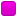June 06, 2023, 12:16:04 pm

###AuthorTopic: Proof by contrapositive  (Read 2110 times) Tweet Share

0 Members and 1 Guest are viewing this topic.

#### jaccs

•• Posts: 7
• Respect: 0##### Proof by contrapositive
« on: February 21, 2022, 12:00:17 pm »
0
Consider the following theorem about prime numbers.
If  (∀ a, b ∈ Z+, p|ab ⇒ p|a or p|b) then p is prime.
Prove the theorem by proving the contrapositive. and we are given hint Put a = p1, where p1 is a prime divisor of p, and b = p/p1

So far have only gotten this far:

contrapositive:
If p is not prime then ∃ a, b ∈ Z+ such that p|ab ⇒ p | a and p | b

any help greatly appreciated

#### RuiAce

• ATAR Notes Lecturer
• Honorary Moderator
• Great Wonder of ATAR Notes
••• Posts: 8814
• "All models are wrong, but some are useful."
• Respect: +2575##### Re: Proof by contrapositive
« Reply #1 on: April 10, 2022, 03:31:54 pm »
+5

To actually prove the contrapositive, use the hint they've given. Suppose that $p$ is composite. Then there exists a prime factor $p_1$ of $p$. However, carefully note that because $p_1$ is a prime divisor of $p$, that $1 < p_1 < p$.

We wish to only prove a "there-exists" statement as you correctly stated, so we may choose our values for $a$ and $b$. Make the specific choice $a=p_1$ and $b=\frac{p}{p_1}$ as they've given in the hint. Then on one hand, it is clearly true that $ab = p$, so the obvious fact that $p\mid p$ implies $p\mid ab$.

On the other hand, we know that $p_1 < p$. Therefore it's impossible that $p \mid p_1$, since no integer is divisible by any integer less than it. Therefore $p\not\mid a$.

Likewise, since $p_1 > 1$, it follows that $\frac{p}{p_1} < \frac{p}{1} = p$. That is, $\frac{p}{p_1}$ is also an integer strictly less than $p$. Hence $p \not\mid \frac{p}{p_1}$, i.e. $p \not\mid b$.

And the contrapositive has now been proven.